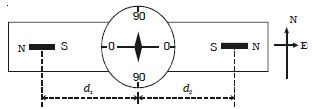# Comparison of Magnetic Moments of Two Bar Magnets in Null Deflection Method

Magnetic Moments of Two Bar Magnets in Null Deflection Method:

The deflection magnetometer is placed in Tan A position (Figure). A bar magnet of magnetic moment M1 and length 2Ɩ1 is placed on one side of the compass box at a distance d1 from the centre of the magnetic needle.The second bar magnet of magnetic moment M2 and length 2Ɩ2 is placed on the other side of the compass box such that like poles of the magnets face each other. The second magnet is adjusted so that the deflection due to the first magnet is nullified and the aluminium pointer reads 0o – 0o.

The distance of the second magnet is x1. The first magnet is reversed pole to pole and placed at the same distance d1. The second magnet is also reversed and adjusted such that the aluminium pointer reads 00 – 00. The distance of the second magnet is x2.

The experiment is repeated by interchanging the magnets. Two more distances x3 and x4 are noted. The mean of x1, x2, x3 and x4 is taken as d2.

As the magnetic fields due to the two bar magnets at the centre of the magnetic needle are equal in magnitude but opposite in direction, B1 = B2

0/4π) * {2M1d1/(d1212)2} = (μ0/4π) * {2M2d2/(d1222)2}

(M1/M2) = [(d1212)2/(d2222)2] * (d2/d1)

If the bar magnets are short, Ɩ1 and Ɩ2 are negligible compared to the distance d1 and d2

so, M1/M2 = d13/d23

It is the equation for magnetic moments of two bar magnets in Null deflection method.